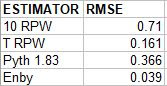## Thursday, April 26, 2018

### Enby Distribution, pt. 6: Accuracy of Enby W% Estimate

In the last post, I demonstrated how one can estimate W% from any runs per game and runs per inning distribution by using the basic principles of how baseball games are decided. This model is simple conceptually, but a bear to implement computationally when compared to the other W% estimators that have been developed by sabermetricians over the last fifty years. As such, it is not a practical tool to use for common sabermetric applications of a winning percentage estimator. If you want to know how many games a team that scores 828 runs and allows 753 runs in a season can expect to win, there are any number of formulas that are better practical options than Enby.

However, it is important to verify that Enby is able to hold its own when estimating W% for normal teams. If it does not work as well as our other tools for normal situations, it will be harder to put any stock in its results when looking at extreme situations.

To check if Enby was up to the challenge, I performed a limited accuracy test based on 1996-2006 data (a sample of 326 teams). This was in no way intended to be a comprehensive accuracy test, but rather one with a sufficiently large sample to determine if Enby can predict normal teams with comparable accuracy to other approaches.

Since I have only calculated Enby distribution parameters at intervals of .05 RG, I rounded all team’s R/G and RA/G to the nearest .05 and used these figures as the inputs for all of the estimators. This ensured that they were all on equal footing, rather than Enby only having some imprecision in terms of the actual R and RA counts. In addition to Enby, I tested four other estimators:

* A simple assumption of 10 RPW
* Tango’s formula that varies RPW by RPG (runs per game for both teams): RPW = .75*RPG + 3. This formula (or at least something very close to it) can be derived by using Pythagenpat.
* Pythagorean with a fixed exponent of 1.83
* Pythagenpat using x = RPG^.28

The resulting RMSE for each estimator (W% RMSE multiplied by 162 for ease of interpretation):The three methods which allow the relationship between runs and wins to vary by scoring context (either by explicitly changing the RPW factor or Pythagorean exponent, or by estimating the scoring distribution as Enby does) come out on top. The linear RPW formula wins here, although the best performer would be Pythagenpat with x = RPG^.29, edging it out at a 3.850 RMSE. Of course, we could also find the coefficients in Tango’s RPW formula that minimize error, and quite possibly push that method back ahead of Pythagenpat.

In any event, the three formulas allowing for customization are close enough that we can safely conclude that none is grossly deficient for the task of estimating W% for normal teams. That means that Enby has passed the first hurdle towards being taken seriously as a model for W% based on average runs scored and allowed.

I also thought it would be interesting to test the RMSE of using each W% estimator to predict Pythagenpat. This is obviously a biased approach, assuming that Pythagenpat is the standard by which other estimators should be compared. The real reason to do this is to see how closely Enby tracks Pythagenpat with normal teams, since Pythagenpat is the closest W% estimator in theory to Enby. Both attempt to dynamically model the relationship between runs and wins; the other approaches, even the dynamic RPW estimator, assume that there is a fixed relationship between runs and wins. We should expect Pythagenpat and Enby to be in general agreement. And they are (RMSE once again multiplied by 162):Enby and Pythagenpat are essentially in lockstep. In fact, the largest discrepancy between the two is for 2002 Braves, who scored 4.40 and allowed 3.50 runs per game (rounded). Pythagenpat expects that such a team would have a W% of .6007, while Enby predicts a .5997 W%, a difference of .15 wins over the course of a season.

The minimum RMSE between Pythagenpat and Enby occurs when the Pythagenpat exponent is dropped slightly to .279 (.026 RMSE). As the exponent varies, the discrepancy increases; with a Pythagenpat exponent of .29, the RMSE is .274.

At this point, I’d like to pause for a moment and change the name of the Enby estimate of W%. This is just for my own sanity as I write and hopefully use these tools in the future, but I want to draw a distinction between the Enby distribution, which is used to estimate the probability of scoring k runs in a game, and the methodology described for estimating W%. I’m a little hesitant to put a name on it, since I haven’t earned that right--the logic is based in reality, not my insight, and has been used by many sabermetricians long before me. Plus, I’m not very good at making up these kinds of names--if you don’t believe me, re-examine the name of the blog.

This methodology is compatible with any means of estimating the probability of scoring k runs a game, whether empirically, through the Enby distribution, solely through the Tango Distribution (as Enby itself borrows from the Tango Distribution), the Weibull distribution (as implemented by Sal Baxamusa or Steven Miller), or any other approach that may be developed in the future. Going forward, I will be referring to this as the Cigol method. As Toirtap can attest, I like to spell things backwards when I am flummoxed. Since the W% estimator is based on simple logic, Cigol it is.